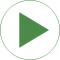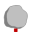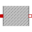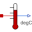# TwoMasses

Simple conduction demo# Information

This information is part of the Modelica Standard Library maintained by the Modelica Association.

This example demonstrates the thermal response of two masses connected by a conducting element. The two masses have the same heat capacity but different initial temperatures (T1=100 [degC], T2= 0 [degC]). The mass with the higher temperature will cool off while the mass with the lower temperature heats up. They will each asymptotically approach the calculated temperature T_final_K (T_final_degC) that results from dividing the total initial energy in the system by the sum of the heat capacities of each element.

Simulate for 5 s and plot the variables
mass1.T, mass2.T, T_final_K or
Tsensor1.T, Tsensor2.T, T_final_degC

# Parameters (1)

T_final_K Value: Type: Temperature (K) Description: Projected final temperature

# Components (5)

mass1 mass2Type: HeatCapacitorType: HeatCapacitorType: ThermalConductorType: TemperatureSensorType: TemperatureSensor